# Hammerstein equation

(diff) ← Older revision | Latest revision (diff) | Newer revision → (diff)

A non-linear integral equation of the type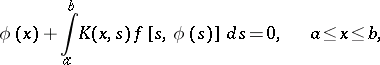whereand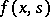are given functions, while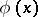is the unknown function. Named after A. Hammerstein , who considered the case whereis a symmetric and positive Fredholm kernel, i.e. all its eigen values are positive. If, in addition, the functionis continuous and satisfies the condition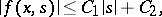where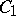and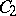are positive constants andis smaller than the first eigen value of the kernel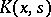, the Hammerstein equation has at least one continuous solution. If, on the other hand,happens to be a non-decreasing function offor any fixedfrom the interval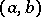, Hammerstein's equation cannot have more than one solution. This property holds also ifsatisfies the condition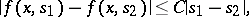where the positive constantis smaller than the first eigen value of the kernel. A solution of the Hammerstein equation may be constructed by the method of successive approximation (cf. Sequential approximation, method of).

How to Cite This Entry:
Hammerstein equation. Encyclopedia of Mathematics. URL: http://encyclopediaofmath.org/index.php?title=Hammerstein_equation&oldid=13494
This article was adapted from an original article by B.V. Khvedelidze (originator), which appeared in Encyclopedia of Mathematics - ISBN 1402006098. See original article